Function Repository Resource:

# GeneralRationalInterpolation

Find a rational interpolation of a function defined parametrically

Contributed by: Wolfram Research
 ResourceFunction["GeneralRationalInterpolation"][{fx,fy},{t,m,n},x,{t1,…,tm+n+1}]] gives the rational polynomial function of x, with numerator order m and denominator order n, that interpolates the curve with x and y coordinates fx and fy generated as a function of t, at the interpolation points t1,t2, …. ResourceFunction["GeneralRationalInterpolation"][{fx,fy},{t,m,n},x,{t,t0,t1}] gives the rational interpolant with the interpolation points chosen automatically from the interval t0 to t1.

## Details and Options

ResourceFunction["GeneralRationalInterpolation"] takes the same options as the resource function RationalInterpolation.

## Examples

### Basic Examples (2)

An approximation to the function whose graph is the upper half-circle:

 In:=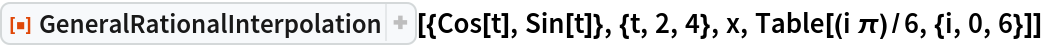Out=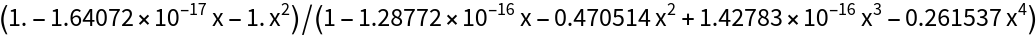The error is quite large near the endpoints:

 In:=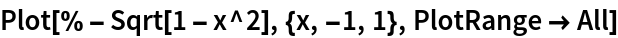Out=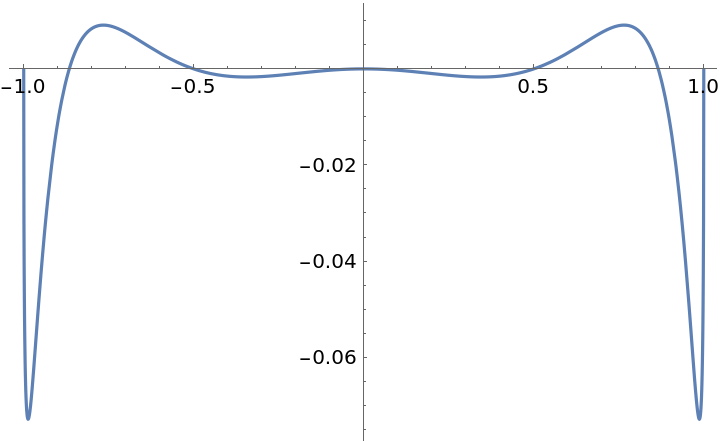Automatically chosen the interpolation points result in a smaller maximum error:

 In:=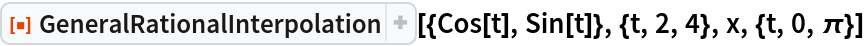Out=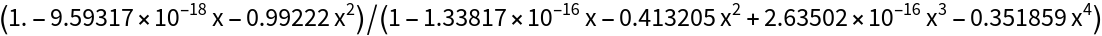In:=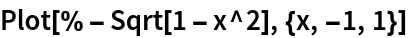Out=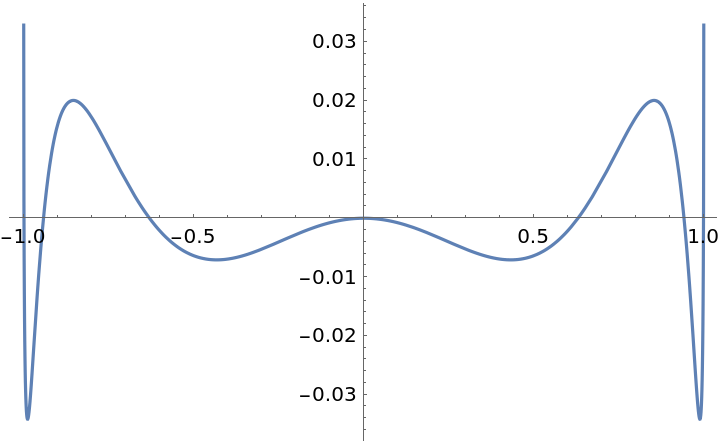## Requirements

Wolfram Language 11.3 (March 2018) or above

## Version History

• 1.0.0 – 28 March 2019